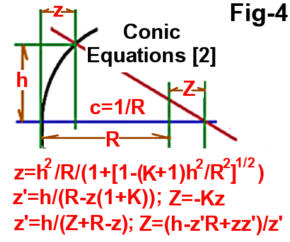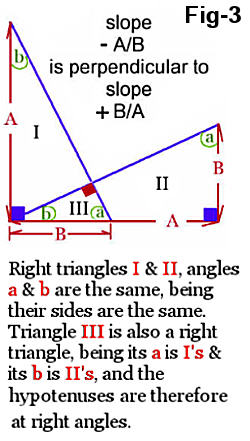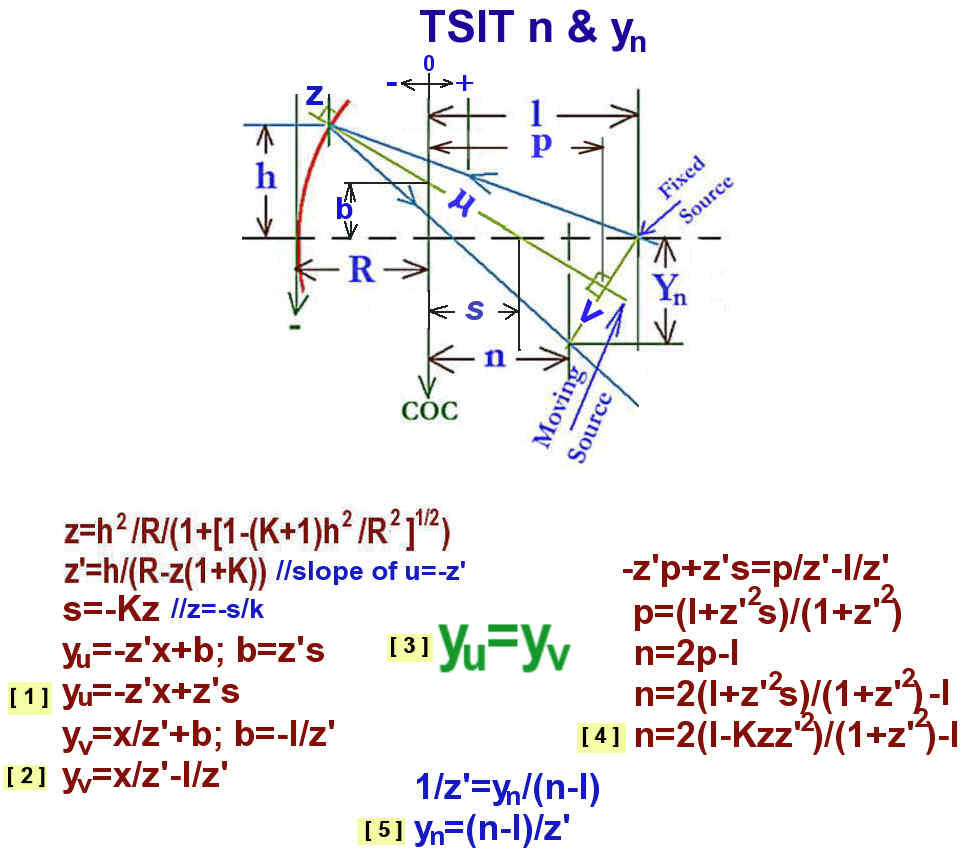Lateral Image Test Overview Computations Setup Baldwin On LIT Contact

Original Slit Image Test Equations

## Conic Equations## SIT Conic Equations## Derivation of SIT Non Linear Algebraic Equations

From the ImageJ slit values, SIT computes the Y's - the differences between each zone pair slit images in inches. SIT nonlinear algebraic equations compute Yi as a function of Z (the Foucaults). Next Excel's Solver is used to find the value of Z such that the difference between Yi and Y is nearly zero. Then Sixtests or FigureXp can be used to compute the mirror surface from the Foucault's.

Mask tests are of two verities, Moving or Fixed Source. The Lateral Wire Test is a Moving Source test. SIT is a Fixed Source test where the camera and source are separated, and as such an exact solution is required rather than an approximation.

To compute the Yi of Fig: Conic fixed source need equations for variables n and Yn of Fig: SIT n and Yn. The derivation for n proceeds using the equation of a straight line y=mx+b where slope m is slope u, the normal to the conic surface (z') and b = z's. From this then, : Yu=-z'x+z's. Next to compute Yv need to know the slope of v (the perpendicular to u), which is the inverse of the slope of u i.e., 1/z' (see proof below).

Then : Yv=X/z'-l/z'. Next find the intersection of lines u and v which is the solution of the simultaneous equations Yv and Yu i.e., : Yv=Yu, which leads to the solution : n=2(l-Kzz'^2)/(1+z'^2)-l. And then knowing n, : Yn=(n-l)/z'.

Now knowing n, and Yn, can solve for Yi of Fig: Conic fixed source if we knew z. From the Conic Equations z = Z/-K, where Z is the Foucault. As stated above, Excel's Solver is used to find the value of Z such that the difference between Yi and Y is nearly zero.

The sum of the angles in a triangle is 180 degrees. The angles in the two large right triangles are the same, being the sides are the same. Angles a and b of the small triangle are the same as that of the large right triangles. Therefore the small triangle is also a right triangle and the slopes (hypotenuses) are at right angles.References
 (1)   Karine and Jean-MarcLecleire, A Manual for Amateur Telescope Making. http://www.willbell.com/tm/atmmanual/index.htm (2)  Conics Optical Shop Testing Edited by Daniel Malacara, Wiley and Sons, 1978, Appendix 1 page 479, 482 Conic approximation Conic equations Conic constant - Schwarzschild constant (3)  LWT J. Francis, "Improved Test Methods for Elliptical and Spherical TCT Mirrors,", ATM J. 14, 34-39, (ISSN 1074-2697). Unfortunately, references to the LWT on the Internet have mostly faded away. Jim Burrows author of Sixtests.exe did mention the LWT. LWT was a variant of the moving source Caustic, where instead of trying to determine a Caustic zone holes focus laterally and longitudinally precisely, the LWT measurements were done manually in the same lateral plane and didn't require a mask. Little judgment was required to determine laterally where a zone ray crossed the lateral plane. The LWT was an improvement over the standard Caustic. However, the required lateral accuracy required was very tight. Given small room temperature and the time to take the measurements, I could not get repeatable LWT results. (4)   Lens mount to CCD spacing    Recommend saving this webpage to your computer, since it has vanished in the past. (5)  Dr. Cuffy's Hartmann2005 - 2023 Bill Thomas This work is licensed under a Creative Commons Attribution-NonCommercial-ShareAlike 4.0 International License.